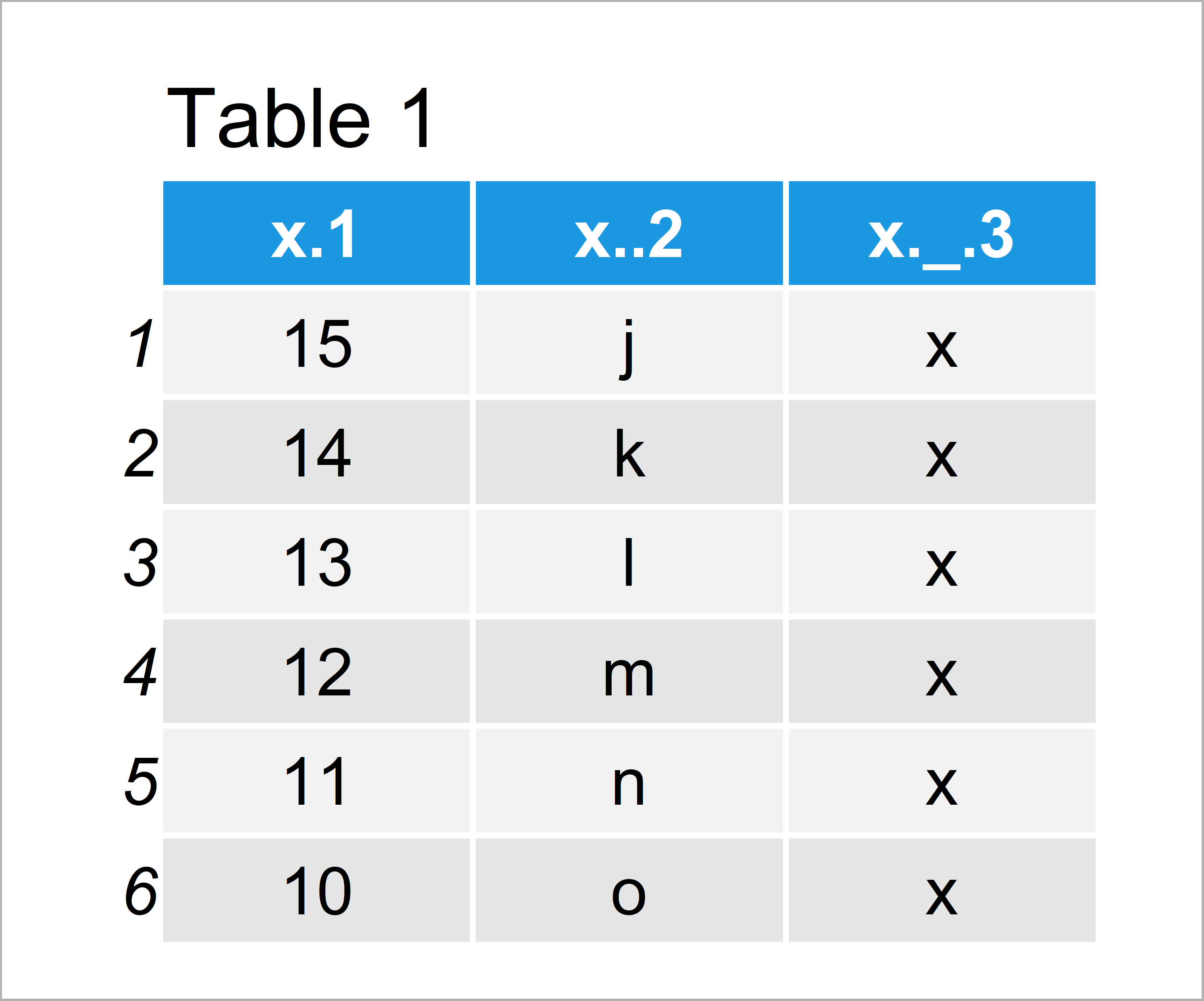# Create Data Frame with Spaces in Column Names in R (Example)

This tutorial illustrates how to retain blanks in the column names of a data frame in the R programming language.

The page looks as follows:

You’re here for the answer, so let’s get straight to the example.

## Example: Hinder data.frame() Function from Converting Blanks in Column Names to Dots

This example illustrates how to keep spaces in the column names when using the data.frame function in R.

First, let’s create a new data frame with default specifications:

```data_dot <- data.frame("x 1" = 15:10, # Create data frame with points "x 2" = letters[10:15], "x _ 3" = "x") data_dot # Print data frame with points```The output of the previous R syntax is visualized in Table 1: We have constructed a data frame where the blanks in the column names have been replaced by points automatically.

So how can we hinder the data.frame function from replacing the blanks in variable names?

Fortunately, the answer is quite simple. We just have to specify the check.names argument of the data.frame function to be equal to FALSE:

```data_space <- data.frame("x 1" = 15:10, # Create data frame with blanks "x 2" = letters[10:15], "x _ 3" = "x", check.names = FALSE) data_space # Print data frame with blanks```After running the previous code the data frame with spaces in the column names shown in Table 2 has been created.

## Video & Further Resources

Do you need further explanations on the R programming codes of this post? Then you could watch the following video tutorial of my YouTube channel. In the video, I illustrate the R code of this article in a live session:

In addition to the video, you might want to read the other articles of this homepage.

To summarize: In this article, I have illustrated how to create a data frame with spaces in the variable names in R programming. Don’t hesitate to let me know in the comments below, in case you have further questions.

Subscribe to the Statistics Globe Newsletter